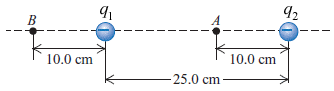# Find the net electric field these charges produce

yaro99

## Homework Statement

Two point charges q1 = -6.15 nC, and q2 = -10.5 nC are separated by 25.0 cm (see figure below).(a) Find the net electric field these charges produce at point A

(b) Find the net electric field these charges produce at point B

(c) What would be the magnitude and direction of the electric force this combination of charges would produce on a proton at A?

E=ke*Q/r2

## The Attempt at a Solution

I got the correct answer for (b), but got an incorrect answer for (a) using the same method.

E = (ke*Q)/(r2)
ƩE = 9*109 * [(6.15*10-9)/(0.152) + (10.5*10-9)/(0.102)] = 11910 N/C to the right

Homework Helper

## Homework Statement

Two point charges q1 = -6.15 nC, and q2 = -10.5 nC are separated by 25.0 cm (see figure below).(a) Find the net electric field these charges produce at point A

(b) Find the net electric field these charges produce at point B

(c) What would be the magnitude and direction of the electric force this combination of charges would produce on a proton at A?

E=ke*Q/r2

## The Attempt at a Solution

I got the correct answer for (b), but got an incorrect answer for (a) using the same method.

E = (ke*Q)/(r2)
ƩE = 9*109 * [(6.15*10-9)/(0.152) + (10.5*10-9)/(0.102)] = 11910 N/C to the right

The electric field is a vector quantity, it has direction. What are the directions of the electric fields due to the individual charges at point (a)?

ehild

yaro99
The electric field is a vector quantity, it has direction. What are the directions of the electric fields due to the individual charges at point (a)?

ehild

Ah, q1 is pointing to the right and q2 to the left, so I subtract the q2 field from the q1 field.

One question: Do I include the +/- charges in my equation? ie. would my values for q1 and q2 in the equation be negative since they are both negative charges?
I got the write answer by just writing the absolute value of my (negative) answer.

edit: completed part (c) with no problems.

Homework Helper
Yes, you need to include the sign of the charge. In case of a negative charge, the electric field points toward the charge. If it is positive, the electric field points away from it. So the electric field due to q1 points to the left, that of q2, points to the right. As the field due to q2 is of greater magnitude, the net electric field points to the right, it is positive.

ehild